# Probabilities For Sums Of Two 6-Sided Dice (Charts & Tables Included)

Rolling two six-sided dice, taking the sum, and examining the possible outcomes is a common way to learn about probability.  Tables and charts are often helpful in figuring out the outcomes and probabilities.

So, what do you need to know about dice probability when taking the sum of two 6-sided dice?  The sum of two 6-sided dice ranges from 2 to 12. A sum of 7 is the most likely to occur (with a 6/36 or 1/6 probability). A sum of 2 (snake eyes) and 12 are the least likely to occur (each has a 1/36 probability). Rolling doubles (the same number on both dice) also has a 6/36 or 1/6 probability.

Of course, a table is helpful when you are first learning about dice probability.

In this article, we’ll look at the probability of various dice roll outcomes and how to calculate them.  We’ll also look at a table to get a visual sense of the outcomes of rolling two dice and taking the sum.

Let’s get started.

## Dice Probability

When we roll a fair six-sided die, there are 6 equally likely outcomes: 1, 2, 3, 4, 5, and 6, each with a probability of 1/6.

When we roll two six-sided dice and take the sum, we get a totally different situation.  There are now 11 outcomes (the sums 2 through 12), and they are not equally likely.

Let’s take a look at the dice probability chart for the sum of two six-sided dice.

### Dice Probability Chart (For The Sum Of Two Six-Sided Dice)

The chart below shows the sums for the 36 possible outcomes when you roll two six-sided dice.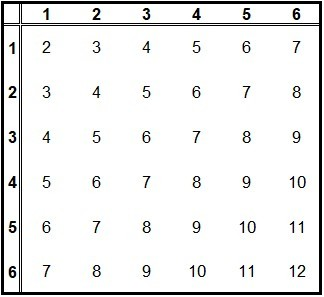This probability chart shows the sums when you roll two 6-sided dice. A 7 is the most likely sum, while 2 and 12 are the least likely sums.

The key to distinguishing between the outcomes (2, 3) and (3, 2) is to think of the dice as having different colors.  For example, think of one die as red, and the other as blue (red outcomes could be the bold numbers in the first column, and blue outcomes could be the bold numbers in the first row, as in the table below).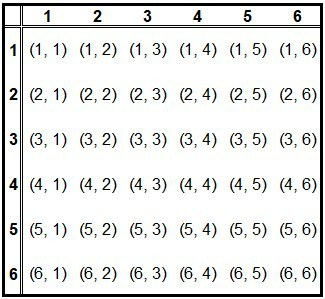This probability chart shows the ordered pairs (RED, BLUE) when you roll two 6-sided dice (RED is in the first column in bold, and BLUE is in the first row in bold).

Then:

• (2, 3) means you rolled a 2 on the red die and a 3 on the blue die
• (3, 2) means you rolled a 3 on the red die and a 2 on the blue die

These two outcomes are different, so (2, 3) in the table above is a different outcome from (3, 2), even though the sums are the same in both cases (2 + 3 = 5).

We can also graph the possible sums and the probability of each of them.  As you can see in the chart below, 7 is the most likely sum, with sums farther away from 7 becoming less likely.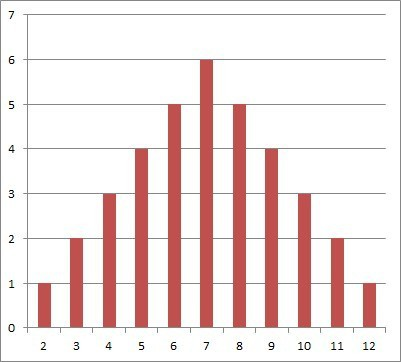This probability chart shows the number of possible ways to achieve each sum (for example, there are 6 ways to get a sum of 7, but only one way to get a sum of 1).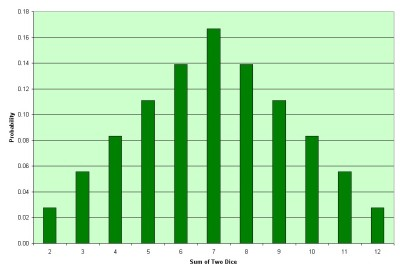This probability chart shows the probability of achieving each sum (for example, there are 6 ways to get a sum of 7, and 36 possible outcomes, so 6/36 / 1/6, or about 0.17, a 17% chance).

Let’s go through the logic of how to calculate each of the probabilities in the able above, including “snake eyes” and doubles.

### Probability Of Rolling Snake Eyes

The probability of rolling snake eyes (two 1’s showing on two dice) is 1/36.

The numerator is 1 because there is only one way to roll snake eyes: a 1 on both dice.

The denominator is 36 (which is always the case when we roll two dice and take the sum).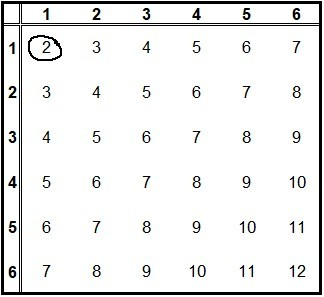There is a 1/36 chance of rolling snake eyes (a 1 on both dice, for a sum of 2).

### Probability Of Rolling Doubles

The probability of rolling doubles (the same number on both dice) is 6/36 or 1/6.

The numerator is 6 because there are 6 ways to roll doubles: a 1 on both dice, a 2 on both dice, a 3 on both dice, a 4 on both dice, a 5 on both dice, or a 6 on both dice.  We can see these outcomes on the  longest diagonal of the table above (from top left to bottom right).

The denominator is 36 (which is always the case when we roll two dice and take the sum).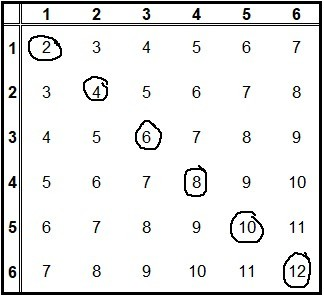The probability of rolling doubles is 6/36 or 1/6 (the outcomes are on the long diagonal from top left to bottom right).

### Probability Of Rolling A 2 With Two Dice

The probability of rolling a 2 with two dice is 1/36.  Note that this is the same as rolling snake eyes, since the only way to get a sum of 2 is if both dice show a 1, or (1, 1).

The numerator is 1 because there is only one way to roll snake eyes: a 1 on both dice.

The denominator is 36 (which is always the case when we roll two dice and take the sum).

### Probability Of Rolling A 3 With Two Dice

The probability of rolling a 3 with two dice is 2/36 or 1/18.

The numerator is 2 because there are 2 ways to roll a 3: (1, 2) – a 1 on the red die and a 2 on the blue die, or (2, 1) – a 2 on the red die and a 1 on the blue die.

The denominator is 36 (which is always the case when we roll two dice and take the sum).

### Probability Of Rolling A 4 With Two Dice

The probability of rolling a 4 with two dice is 3/36 or 1/12.

The numerator is 3 because there are 3 ways to roll a 4: (1, 3), (2, 2), and (3, 1).

The denominator is 36 (which is always the case when we roll two dice and take the sum).

### Probability Of Rolling A 5 With Two Dice

The probability of rolling a 5 with two dice is 4/36 or 1/9.

The numerator is 4 because there are 4 ways to roll a 5: (1, 4), (2, 3), (3, 2), and (4, 1).

The denominator is 36 (which is always the case when we roll two dice and take the sum).

### Probability Of Rolling A 6 With Two Dice

The probability of rolling a 6 with two dice is 5/36.

The numerator is 5 because there are 5 ways to roll a 6: (1, 5), (2, 4), (3, 3), (4, 2), and (5, 1).

The denominator is 36 (which is always the case when we roll two dice and take the sum).

### Probability Of Rolling A 7 With Two Dice

The probability of rolling a 7 with two dice is 6/36 or 1/6.  Note that this is the highest probability of any sum from 2 to 12, and thus the most likely sum when you roll two dice.

The numerator is 6 because there are 6 ways to roll a 7: (1, 6), (2, 5), (3, 4), (4, 3), (5, 2), and (6, 1).

The denominator is 36 (which is always the case when we roll two dice and take the sum).

### Probability Of Rolling A 8 With Two Dice

The probability of rolling an 8 with two dice is 5/36.

The numerator is 5 because there are 5 ways to roll an 8: (2, 6), (3, 5), (4, 4), (5, 3), and (6, 2).

The denominator is 36 (which is always the case when we roll two dice and take the sum).

### Probability Of Rolling A 9 With Two Dice

The probability of rolling a 9 with two dice is 4/36 or 1/9.

The numerator is 4 because there are 4 ways to roll a 9: (3, 6), (4, 5), (5, 4), and (6, 3).

The denominator is 36 (which is always the case when we roll two dice and take the sum).

### Probability Of Rolling A 10 With Two Dice

The probability of rolling a 10 with two dice is 3/36 or 1/12.

The numerator is 3 because there are 3 ways to roll a 10: (4, 6), (5, 5), and (6, 4).

The denominator is 36 (which is always the case when we roll two dice and take the sum).

### Probability Of Rolling A 11 With Two Dice

The probability of rolling an 11 with two dice is 2/36 or 1/18.

The numerator is 2 because there are 2 ways to roll an 11: (5, 6) and (6, 5).

The denominator is 36 (which is always the case when we roll two dice and take the sum).

### Probability Of Rolling A 12 With Two Dice

The probability of rolling a 12 with two dice is 1/36.

The numerator is 1 because there is only one way to roll 12: a 6 on both dice, or (6, 6).

The denominator is 36 (which is always the case when we roll two dice and take the sum).